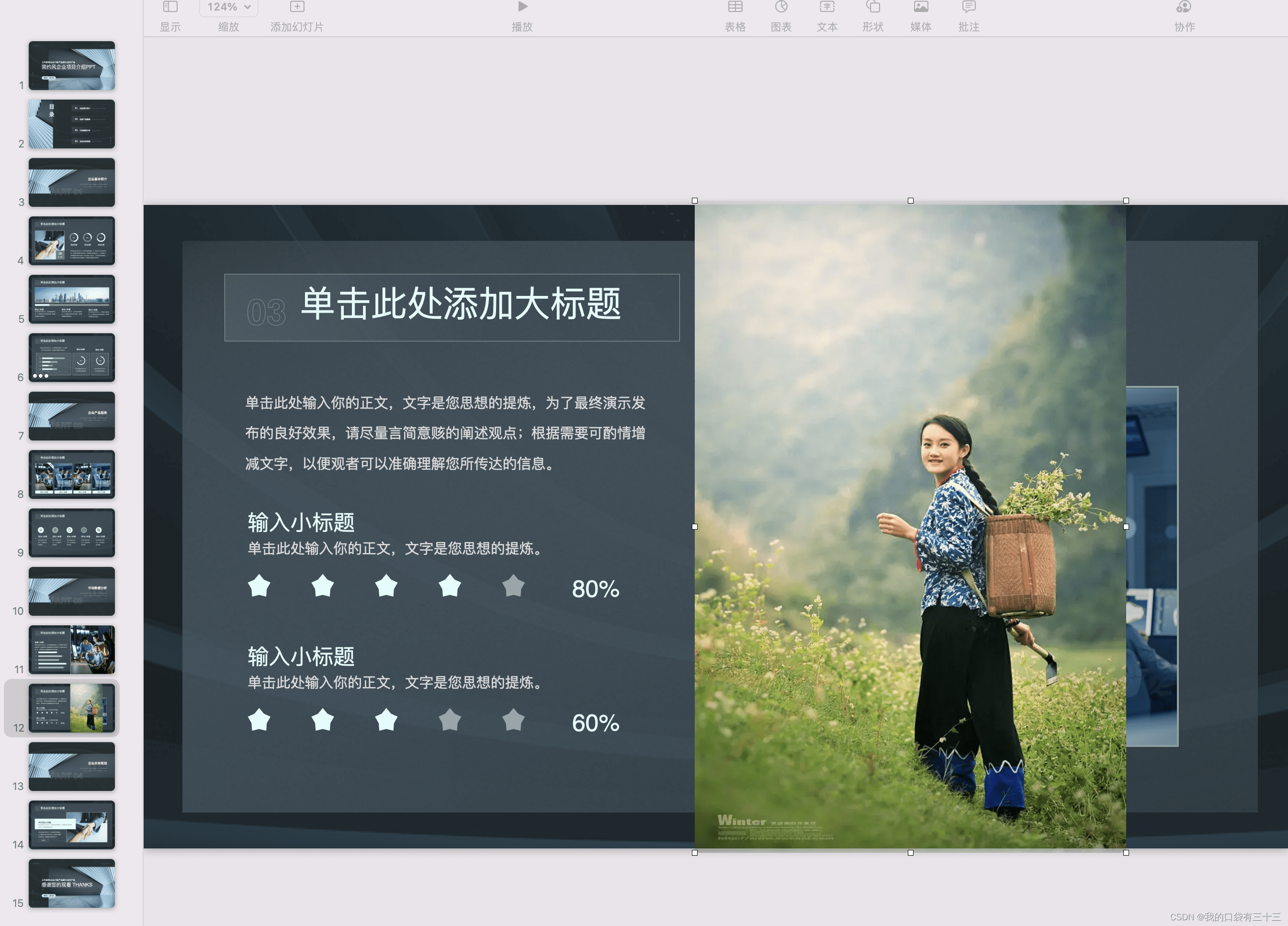# 用python抠图

GitHub上有个好完的项目，rembg## 1、安装

CPU support:

``````pip install rembg
``````

GPU support:

``````pip install rembg[gpu]
``````

## 2、使用

### 2.1 以命令行方式使用

#### 2.1.1、在命令行中，切换到使用的虚拟环境，我的虚拟环境起名叫py310

``````conda activate py310
``````

#### 2.1.2、开始抠图

``````# 1、扣本地的图
rembg i path/to/input.png path/to/output.png
# 2、扣网上的图
curl -s http://input.png | rembg i > output.png
# 3、扣整个文件夹的图
rembg p path/to/input path/to/output
``````### 2.2以服务的方式使用

#### 2.2.1 官方网页版

``````# 在虚拟环境中，敲以下命令：
rembg s
``````get方式适用于网上的URL地址
post方式适合文件流，这里我们使用post的方式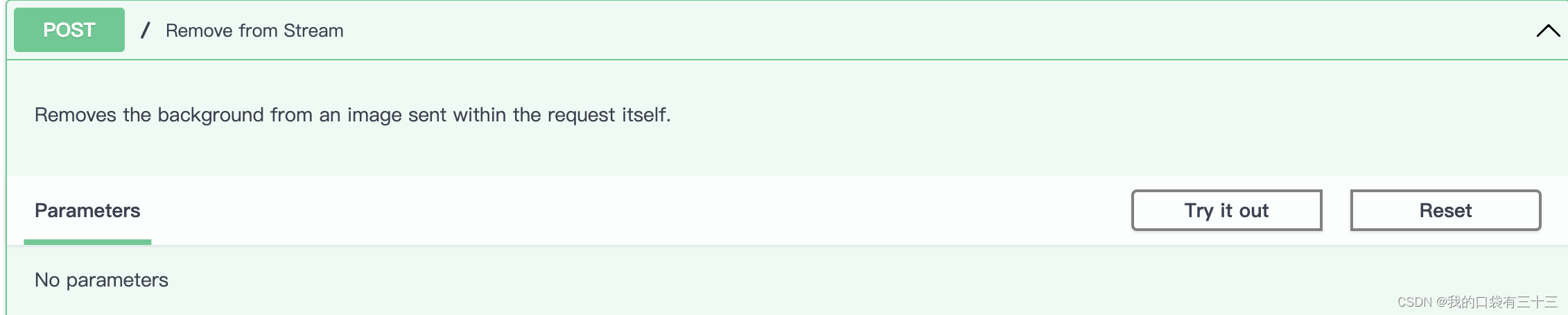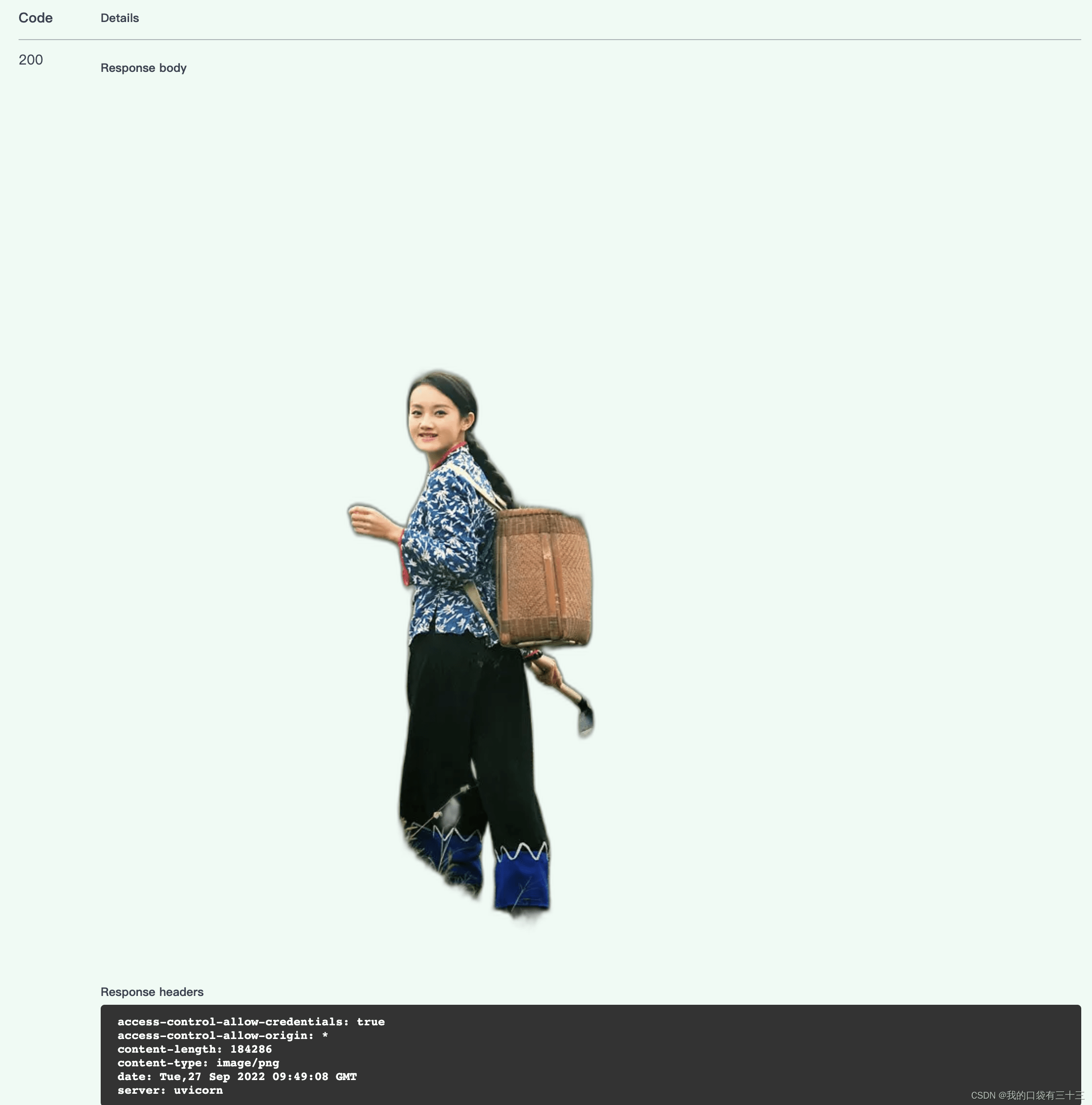#### 2.2.2 自己做网页用

``````<form
action="http://http://0.0.0.0:5000"
method="post"
enctype="multipart/form-data"
>
<input type="file" name="file" />
</form>
``````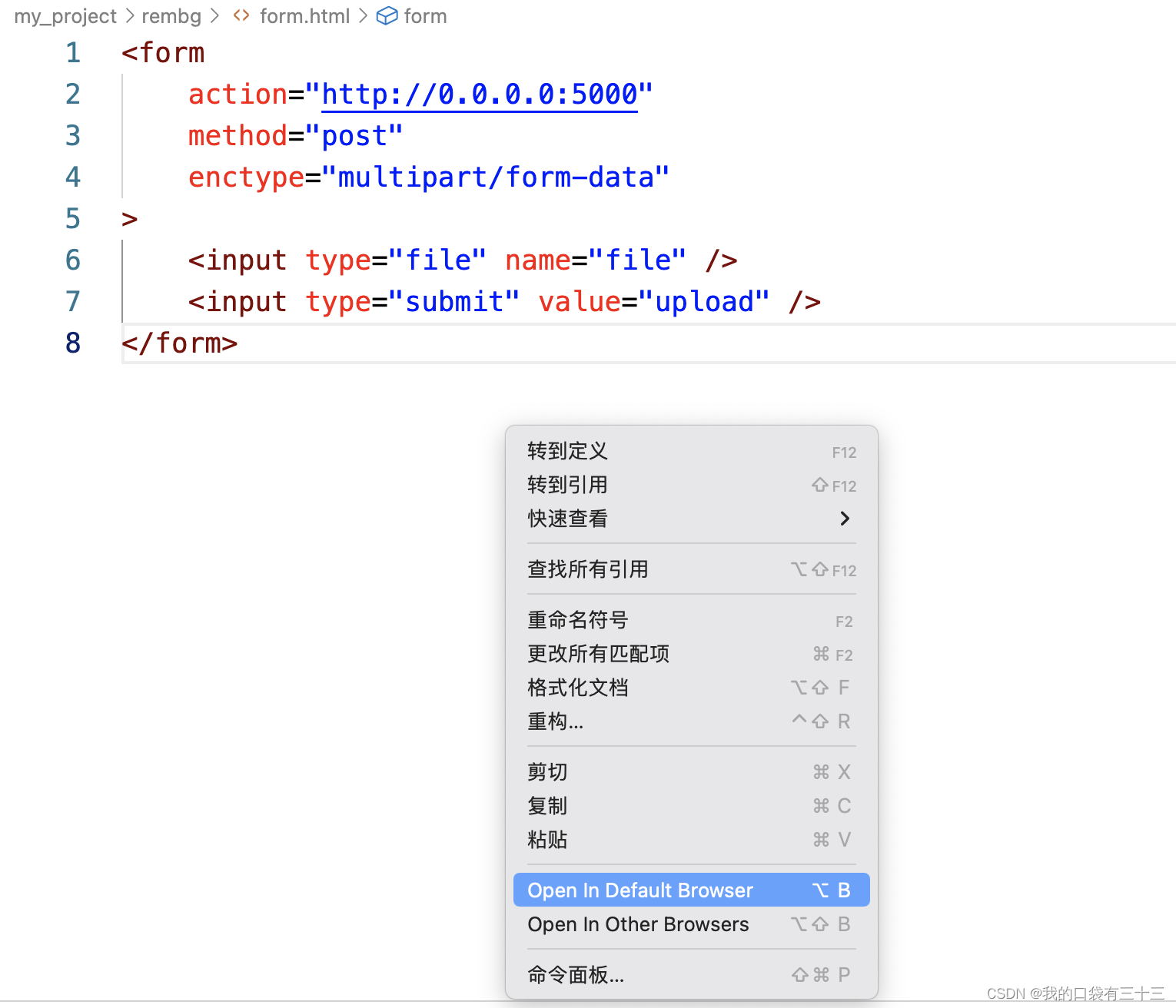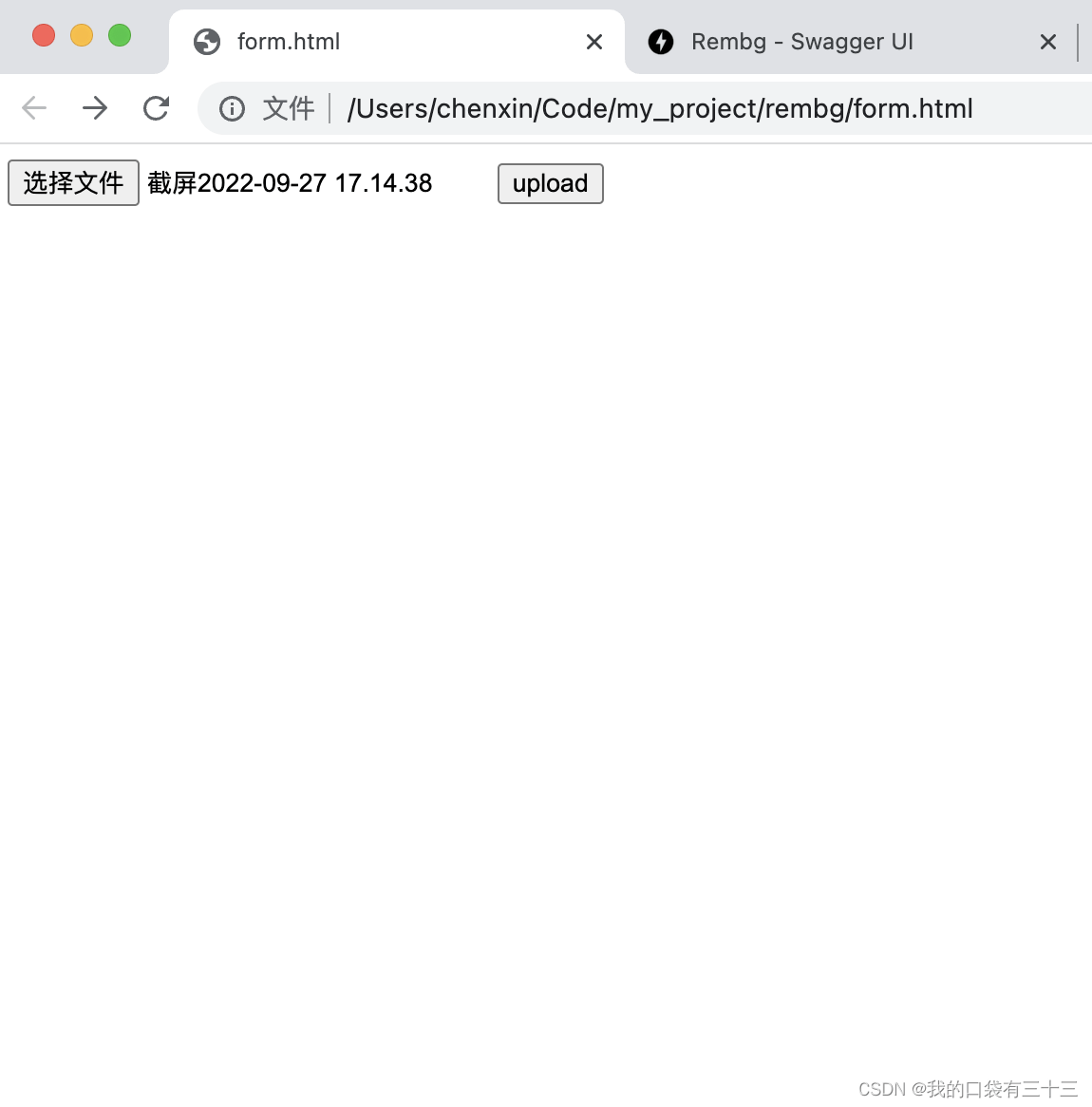### 2.3以一个python库的方式使用

#### 2.3.1 以文件流的方式

Input and output as bytes

``````from rembg import remove

input_path = 'input.png'
output_path = 'output.png'

with open(input_path, 'rb') as i:
with open(output_path, 'wb') as o:
output = remove(input)
o.write(output)
``````

#### 2.3.2 用PIL图像的方式

Input and output as a PIL image

``````from rembg import remove
from PIL import Image

input_path = 'input.png'
output_path = 'output.png'

input = Image.open(input_path)
output = remove(input)
output.save(output_path)
``````

#### 2.3.3 甚至于用numpy array

Input and output as a numpy array

``````from rembg import remove
import cv2

input_path = 'input.png'
output_path = 'output.png'

output = remove(input)
cv2.imwrite(output_path, output)
``````

2.4以docker的方式使用（这个以后试试）

``````docker run -p 5000:5000 danielgatis/rembg s
``````